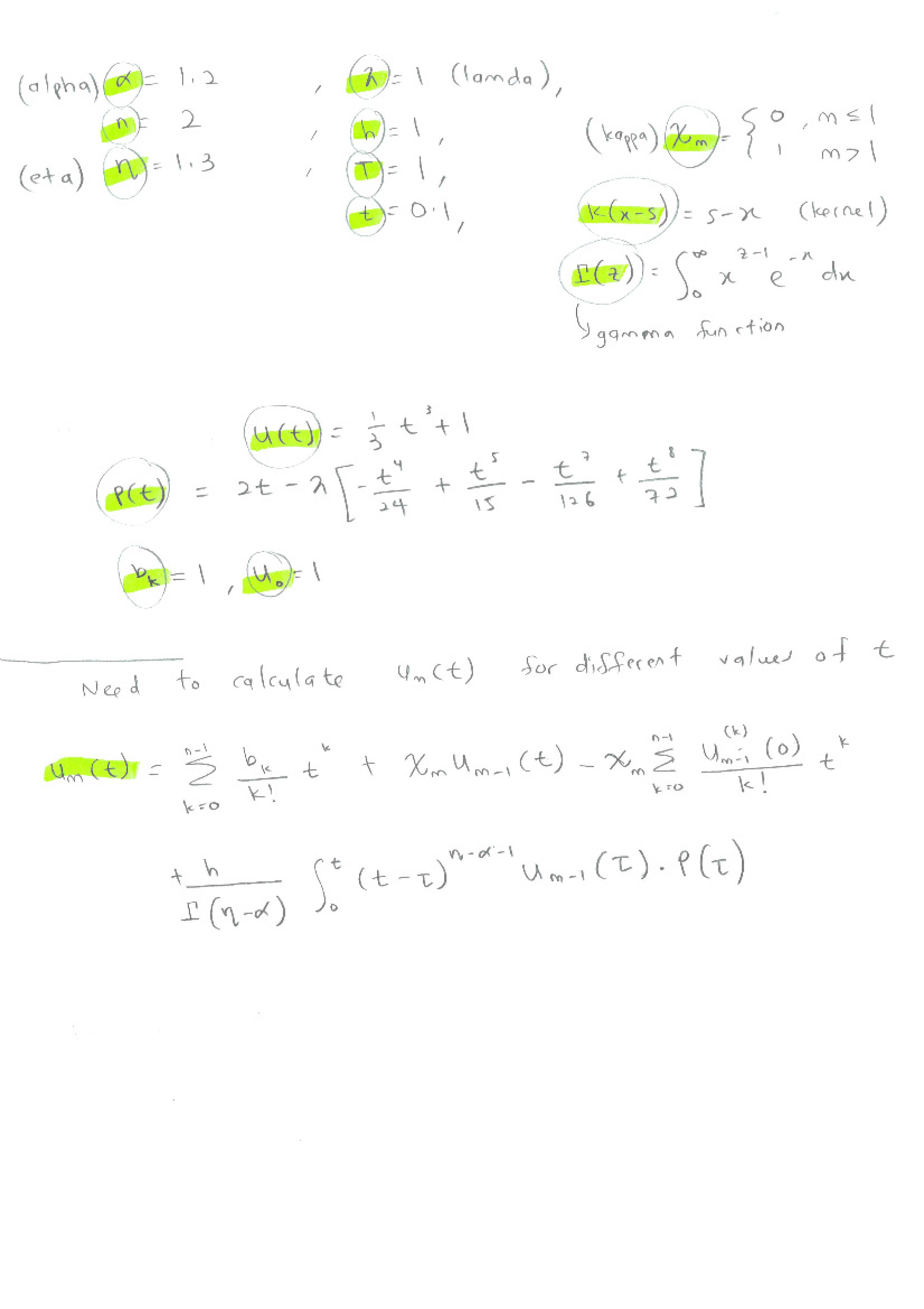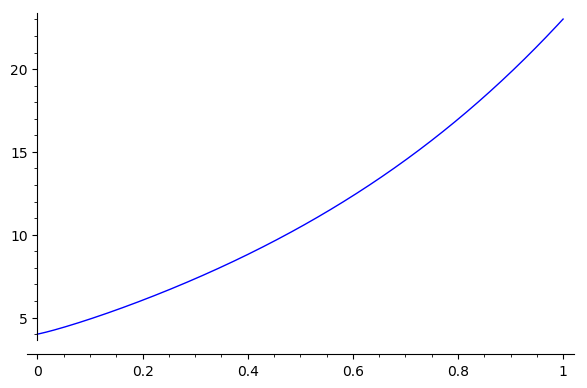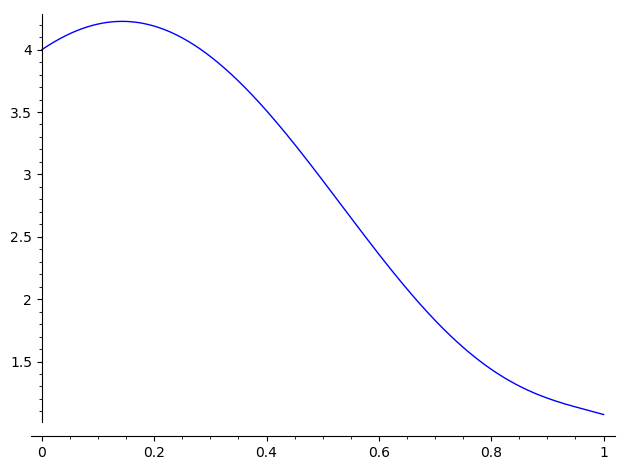# for-loop not computing the values

Hi. I am quite new to using phyton is SAGE, but what I did here is that I have tried my best to come up with the code based on reading multiple notes on SAGE.The values of alpha, n, eta, lambda, h, T, t, kappa, k(x-s) and gamma values are given.

I have tried to do the coding from scratch and this is what I have obtained:

from __future__ import print_function
alpha = 1.2
n = 2
eta = 1.3
lamda = 1
h = 1
T = 1
t = 0.1

h, t, x, s = var('h t x s')
U[t] = 1/3*t^3+1
P[t] = 2*t-lamda*(-t^4/24+t^5/15-t^7/126+t^8/72)
b[k] = 1

def X(m):
if m <= 1:
return 0
else:
return 1

k[x,s]=s-x
gamma[z]=integrate(x^(z-1)*e^(-x),x, 0, infinity)
u=1

for m in range(1,4):
u[m] = sum(b[k]/factorial(k)*t^k,k,0,n-1) + X[m]*u[m-1]-X[m]*sum(𝚍𝚎𝚛𝚒𝚟𝚊𝚝𝚒𝚟𝚎(u[m-1],t,k)/factorial(k)*t^k,k,0,n-1)+h/gamma[eta-alpha]*integrate((t-tau)^(eta-alpha-1)*u[m-1].subs(t=tau)*P[t].subs(t=tau),tau, 0, t); print(u[m].full_simplify())

UU=u+u+u+u:


What I am trying to achieve here is the values of u_1, u_2, u_3, u_4. Then calculate UU = u_1 + u_2 + u_3 + u_4.

At the moment, I am not getting the answer. Not sure what is causing the error. It took me so much time and effort to come up with this from scratch, hope someone can help me indicate what's wrong with this coding. Thank you in advance.

edit retag close merge delete

Hello, @Sha! I have corrected some errors in your code. However, there seems to be a couple of problems in Sage. On one side, the last integral in the definition of u[m] can't be computed directly, so I had to use a couple of auxiliary variables. This helped, but the loop only computes one iteration, and then freezes until Sage gives up.

I suppose there are some optimizations that can be carried out in the loop. Let me try for a couple of days and I'll let you know. Do you have a deadline for this?

1
1

U and P look like functions, so you should use parentheses when you define them. b and u look like lists or dictionaries or vectors, and you need to define them as such before setting entries in them. u=1 will give an error, for example, unless you have first done something like u={}. Also, note that for m in range(1,4): will loop over values m=1, m=2, and m=3, but not m=4.

@dsejas Hi diego. thank you for your reply. I am actually trying to figure out the formula too.. just to check if there's anything wrong with it. I don't have a deadline with this. Thank you for your help. Let me too re check the calculation manually.

@johnpalmieri thank you for the feedback John. I really appreciate it.

Sort by » oldest newest most votedIn your handwritten note, one sees that $u_0=1$ and that, for $n\geq 1$,

$$u_n(t)=\sum_{k=0}^{n-1} \frac{b_k}{k!} t^k + \chi_n u_{n-1}(t) -\chi_n \sum_{k=0}^{n-1} \frac{u_{n-1}^{(k)}(0)}{k!} t^k +\frac{h}{\Gamma(\eta-\alpha)} \int_0^t (t-\tau)^{n-\alpha-1} u_{n-1}(\tau) P(\tau) \,d\tau.$$

This expression can be rewritten as follows:

$$u_n(t)=\sum_{k=0}^{n-1} \frac{b_k}{k!} t^k + \chi_n \bigl(u_{n-1}(t) -q_{n-1}(t)\bigr) +\frac{h}{\Gamma(\eta-\alpha)} I_n(t),$$

where $q_{n-1}$ is the MacLaurin polinomial of $u_{n-1}$ of order $n-1$ and

$$I_n(t) = \int_0^t (t-\tau)^{n-\alpha-1} u_{n-1}(\tau) P(\tau) \,d\tau.$$

You have defined $\chi_n$ as $\chi_n=1$ for $n\geq 2$, as well as $\chi_1=0$ to avoid the term $u_0(t) -q_0(t)$ in the expression of $u_1$. However, since $u_0(t)=1$, it is obvious that $q_0(t)=1$. Hence $u_0(t) -q_0(t)=0$. This implies that, in fact, you do not need $\chi_n$.

Let us consider $I_n(t)$. I think that $n-\alpha-1$ should be greater than $-1$ for this integral to be convergent. Since $\alpha=1.2$, there is a problem in the case $n=1$.

Finally, what is the sense of $U(t)=t^3/3+1$? This function does not appear in the computation of any $u_n$.

Taking all the above facts into account, I have rewritten your code. I do not define $\chi_n$ (not needed), I ignore $U(t)$ and I take a value of $\alpha$ so that $n-\alpha-1>-1$ for any $n$, such as $\alpha=0.8$. It seems that SageMath does not like, at least in this case, float numbers as exponents, so I write $\alpha=8/10$ instead of $\alpha=0.8$. Here it is the code:

var("t,tau")
lamda = 1
P(t) = 2*t - lamda*(-t^4/24+t^5/15-t^7/126+t^8/72)
h = 1
eta = 1.3
alpha = 8/10
nmax = 4
b = nmax*

forget()
assume(t>0)
u = [SR(1).function(t)]
for n in [1..nmax]:
un = sum(b[k]*t^k/factorial(k) for k in [0..n-1])
un += u[n-1](t) - taylor(u[n-1](t), t, 0, n-1)
un += (h/gamma(eta-alpha))*integrate((t-tau)^(n-alpha-1)*u[n-1](tau)*P(tau),tau,0,t)
u.append(un.function(t))

UU(t) = sum(u[k](t) for k in [1..nmax])
forget()


Observe that I introduce the variable nmax, for the case being you want to compute more functions $u_n$. Likewise, SageMath needs some help to integrate, so I added the assumption that $t>0$. The forget commands delete the effect of any assume command; thus, SageMath does not get confused by any previous assume you could been using before, and stops using the assumption $t>0$ once $UU$ is computed.

The main loop provides a list with the functions $u_0$, $u_1$,...,$u_{nmax}$. They are symbolic callable functions, so to evaluate, say, $u_3$ at $t=0.5$, you simply write u(0.5).

The code also defines $UU$. You can show it

sage: show(UU(t))
[Very large output]


evaluate it at, say, $t=0.9$

sage: UU(0.9).n()
19.7969629979363


or plot it

sage: plot(UU(t), (t,0,1))
[Long time]more

Hello, @Sha! I am quite fascinated by @Juanjo's answer. I suggest you to take a careful look at his reasoning and derivations. However, I would also like to try to answer your post, since I got different results (and formulation, see below), and I have some tiny improvements.

But first, I would like to point out that I separated every term in your definition of u[m], and I experimented with each one of them. I found out that the computation stops after the first iteration do to the integral

$$\int_0^t(t-\tau)^{\eta-\alpha-1}u_{m-1}(\tau)P(\tau)\;d\tau$$

The problem is that $\eta-\alpha-1=-0.9$, and Sage seems to be unwilling to compute the integral in the second iteration in that case. (I really don't know why!) I tried to mitigate this problem by computing the indefinite integral first, and that helped a little bit. That's the reason why I used the aux (the indefinite integral) and aux1 (the definite integral) temporary variables in the code that I sent you in my previous comment. However, it didn't help much.

Once again, I don't know why, but using rational numbers instead of floating point numbers did work. (This is also pointed out by @Juanjo's answer.) As a consequence, I have replaced the definitions of $\alpha$ and $\eta$ using rational numbers:

alpha = 6/5
eta = 13/10


That tells Sage to use rational numbers, not floating point numbers, and that should avoid the problems with the integral.

Anyway, in the following code, I have deleted the kernel k (since you don't use it in your calculations). I have also deleted your definition of the gamma function, since it is predefined in Sage. On the other hand, notice that

$$\sum_{k=0}^{n-1}\frac{b_k}{k!}t^k$$

and

$$\sum_{k=0}^{n-1}\frac{u_{m-1}^{(k)}}{k!}t^k$$

are the Taylor Polynomials of degree $n-1$ in $t=0$ of the functions $b_ke^t$ and $u_{m-1}(t)$.

Now, I think, @Juanjo made a mistake when he said that $\chi$ is unnecessary. I think the problem is that he wrote the original formula (which was written in terms of $m$ and $n$) in terms of just $n$. If I understood correctly, $n$ is a constant and $m$ is your iteration variable (am I right?). If that is the case, the $\chi$ is needed in front of u[m-1], but not in front of your second summation, which is the Taylor Polynomial for $u_{m-1}(t)$ (as I just explained), which turns out to be zero for $m=1$, since $u_0(t)=1$. However, notice that $\chi(m)$ is equivalently defined as $\operatorname{sign}(m-1)$, so I used sign(m-1).

Finally, Sage asked me to specify if $t>0$ or $t<0$ (so it can compute the integral). I assumed $t>0$. That's the command assume(t>0).

If I did everything right, the following code should work:

from __future__ import print_function

NUMBER_OF_ITERATIONS = 4

alpha = 6/5
n = 2
eta = 13/10
lamda = 1
h = 1
T = 1

var('h t x s j tau')
U(t) = 1/3*t^3+1
P(t) = 2*t-lamda*(-t^4/24+t^5/15-t^7/126+t^8/72)
b_k = 1

u = vector(SR, NUMBER_OF_ITERATIONS)
u = 1
U = u
assume(t>0)
for m in range(1, NUMBER_OF_ITERATIONS):
u[m] = b_k*taylor(e^t, t, 0, n-1) + sign(m-1)*u[m-1] - taylor(u[m-1], t, 0, n-1) + h/gamma(eta-alpha)*integrate((t-tau)^(eta-alpha-1)*(u[m-1].subs(t=tau))*P(tau),tau, t, 0); print(u[m])
U += u[m]

forget()
show(U(t))
print('U(0.1) =', N(U(t=0.1,h=1)))
plot(U(t).subs(h=1), t, 0, 1)I hope this helps! As always, if the answer needs to be modified or re-explained you can ask as a comment or send me a mail.

more

1

I am really worried that $m$ and $n$ could be the same variable, could you confirm that $n=2$ is a constant and $m$ is the iteration variable?

1

It is often difficult to distinguish between $n$ and $m$ in a handwritten text. Perhaps this has been my case. Anyway, it would be convenient that @Sha clarifies the formulae, rewriting them with LaTeX... or a more careful calligraphy ;-))

1

I agree, @Juanjo. It took me some time to understand the formulas, and I am not completely sure that I understood them correctly.

On the other hand, I run some more experiments, and Sage seems unwilling to compute more than 4 iterations of the recurrence formula. The problem continues to be the integral. Apparently, it grows very fast, and Sage has difficulties trying to compute the result. I wonder why.

Quite interestingly, if I remove the integral, the series converges to $t+1$.